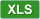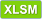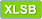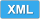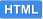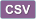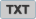﻿ Convert CSV to Excel file in C++ | EasyXLS Guide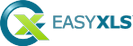# How to convert CSV file to Excel in C++

 ``````/* --------------------------------------------------------------------- * Tutorial 39 * * This tutorial shows how to convert CSV file to Excel in C++. The * CSV file generated by Tutorial 30 is imported, some data is modified * and after that is exported as Excel file. * --------------------------------------------------------------------- */ Click here to see the Excel file #include "stdafx.h" #include "EasyXLS.h" #include int _tmain(int argc, _TCHAR* argv[]) { printf("Tutorial 39\n----------\n"); HRESULT hr; // Initialize COM hr = CoInitialize(0); // Use the SUCCEEDED macro and get a pointer to the interface if (SUCCEEDED(hr)) { // Create a pointer to the interface used to import/export Excel files EasyXLS::IExcelDocumentPtr workbook; hr = CoCreateInstance(__uuidof(EasyXLS::ExcelDocument), NULL, CLSCTX_ALL, __uuidof(EasyXLS::IExcelDocument), (void**) &workbook) ; if (SUCCEEDED(hr)){ // Import CSV file printf("\nReading file: C:\\Samples\\Tutorial30.csv\n"); if (workbook->easy_LoadCSVFile("C:\\Samples\\Tutorial30.csv")) { // Set worksheet name workbook->easy_getSheetAt(0)->setSheetName("First tab"); // Add new worksheet and add some data in cells (optional step) workbook->easy_addWorksheet_2("Second tab"); EasyXLS::IExcelWorksheetPtr xlsSecondTab = (EasyXLS::IExcelWorksheetPtr)workbook->easy_getSheetAt(1); EasyXLS::IExcelTablePtr xlsTable = xlsSecondTab->easy_getExcelTable(); xlsTable->easy_getCell_2("A1")->setValue("Data added by Tutorial39"); char* cellValue = (char*)malloc(11*sizeof(char)); char* columnNumber = (char*)malloc(sizeof(char)); for (int column=0; column<5; column++) { strcpy_s(cellValue, 6, "Data "); _itoa_s(column+ 1, columnNumber, 2, 10); strcat_s(cellValue, 10, columnNumber); xlsTable->easy_getCell(1, column)->setValue(cellValue); } // Export Excel file printf("Writing file C:\\Samples\\Tutorial39.xls."); workbook->easy_WriteXLSFile("C:\\Samples\\Tutorial39.xls"); // Confirm conversion of CSV to Excel _bstr_t sError = workbook->easy_getError(); if (strcmp(sError, "") == 0){ printf("\nFile successfully created. Press Enter to Exit..."); } else{ printf("\nError encountered: %s", (LPCSTR)sError); } } else { printf("\nError reading file C:\\Samples\\Tutorial30.csv %s\n", (LPCSTR)((_bstr_t)workbook->easy_getError())); } // Dispose memory workbook->Dispose(); } else{ printf("Object is not available!"); } } else{ printf("COM can't be initialized!"); } // Uninitialize COM CoUninitialize(); _getch(); return 0; } ``````
C++ and .NET Framework
This tutorial is valid for C++ applications that cannot use .NET Framework. It is recommended, for better performances, that if the C++ application already uses or can use .NET Framework to make use of this similar code sample.

For methods with same name but different parameters, only the first method overload retains the original name. Subsequent overloads are uniquely renamed by appending to the method name '_2', '_3', etc (method, method_2, method_3), an integer that corresponds to the order of declaration that can be found in EasyXLS.h, a file that comes with EasyXLS installation.

#### EasyXLS Excel libraries: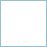##### Excel Library for C++
full COM+ version to import, export or convert Excel files#### File formats:

MS Excel 97
MS Excel 2003
MS Excel 2007
MS Excel 2010
MS Excel 2013
MS Excel 2016
MS Excel 2019